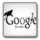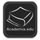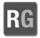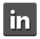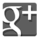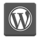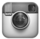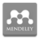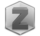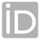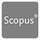You are in:Home/Publications

### Dr. amal foad abdelaziz soliman :: Publications:

Research Fields
 No Research Fields
 Number of publications is 6
 Title 1-FOURTH AND SIXTH ORDER COMPACT FINITE DIFFERENCE SCHEMES FOR PARTIAL INTEGRO-DIFFERENTIAL EQUATIONS 2-THE NUMERICAL SOLUTION OF LNTEGRO-PARTIAL DIFFERENTIAL EQUATIONS WITH SIXTH-DEGREE B-SPLINE FUNCTIONS 3-On The Numerical Solution of Partial integro-differential equations 4-A comparison of Semi-analytical Methods for Solving Partial Integro- Differential Equations 5-Compact Finite Difference Schemes for Solving a Class of Weakly- Singular Partial Integro-differential Equations 6-Compact Finite Difference Schemes for Partial integrodifferential Equations